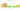RON

Romanian Leu

how has the value of the currency changed in the last year?USD 1 = RON 4.323

How much is10 US dollars worth inRomanian lei?
At the current exchange rate, 10 US dollars is worth 43.23 Romanian lei
How much is50 US dollars worth inRomanian lei?
At the current exchange rate, 50 US dollars is worth 216.15 Romanian lei
How much is100 US dollars worth inRomanian lei?
At the current exchange rate, 100 US dollars is worth 432.3 Romanian lei
How much is500 US dollars worth inRomanian lei?
At the current exchange rate, 500 US dollars is worth 2,161.5 Romanian lei
How much is2,000 US dollars worth inRomanian lei?
At the current exchange rate, 2,000 US dollars is worth 8,646 Romanian lei
How much is10 US dollars worth inRomanian lei?
At the current exchange rate, 10 US dollars is worth 43.23 Romanian lei
How much is50 US dollars worth inRomanian lei?
At the current exchange rate, 50 US dollars is worth 216.15 Romanian lei
How much is100 US dollars worth inRomanian lei?
At the current exchange rate, 100 US dollars is worth 432.3 Romanian lei
How much is500 US dollars worth inRomanian lei?
At the current exchange rate, 500 US dollars is worth 2,161.5 Romanian lei
How much is2,000 US dollars worth inRomanian lei?
At the current exchange rate, 2,000 US dollars is worth 8,646 Romanian lei